# Problem 1 (15%)¶

Install newest release of TensorFlow 1.4 on the operating system of your choice. Use installation instructions on https://www.tensorflow.org site and instructions on https://github.com/tensorflow/tensorflow. If you know what you are doing install TensorFlow for GPU. Otherwise, install TensorFlow for CPU. Use attach Jupyter notebook: 0_test_install.ipynb to demonstrate that TensorFlow is properly installed. Please document all installation steps including the version of Python you are using.

### Installation¶

I'm using python 2.7 and TensorFlow 1.4 for the following analysis. The software is installed in a virtual environment. The following code is installing the software on my ec2 instance:

Create environment

conda create -n tensorflow python=2.7 anaconda
source activate tensorflow


Install pip

sudo apt install -y python-pip


Install Tensorflow and pandas

pip install --ignore-installed --upgrade tensorflow
pip install pandas


Install conda packages

conda install matplotlib
conda install nb_conda


### Check installation¶

In :
import tensorflow as tf
hello = tf.constant('It works!')
sess = tf.Session()
print(sess.run(hello))

It works!

In :
# Check that you have a recent version of TensorFlow installed, >= 0.12.0rc0
print("You have version %s" % tf.__version__)

You have version 1.4.0

In :
%matplotlib inline
import pylab
import numpy as np

# create some data using numpy. y = x * 0.1 + 0.3 + noise
x_train = np.random.rand(100).astype(np.float32)
noise = np.random.normal(scale=0.01, size=len(x_train))
y_train = x_train * 0.1 + 0.3 + noise

# plot it
g = pylab.plot(x_train, y_train, '.')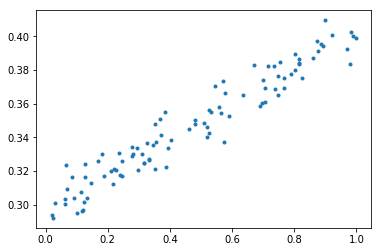# Problem 2. (25%)¶

Construct a simple neural network (a network of logistic units) which will implement (X1 XOR X2) AND X3 function. Choose weights (θ_i-s) of all dendritic inputs and bias inputs. Demonstrate that your network works by presenting the truth table. Present your network by a simple graph. You can produce the graph in any way convenient including pan and paper.

### i) XOR Problem¶

We're starting solving the X1 XOR X2 problem. In the case of x1 XOR x2, the value is true if one of the values is true. We can construct a truth table that look like the following:

X1 X2 b(x)
0 0 0
0 1 1
1 0 1
1 1 0

A network would look like the following:

In :
## This is for an png image
from IPython.display import Image

fig = Image(filename=('img/xor.png'))
fig

Out: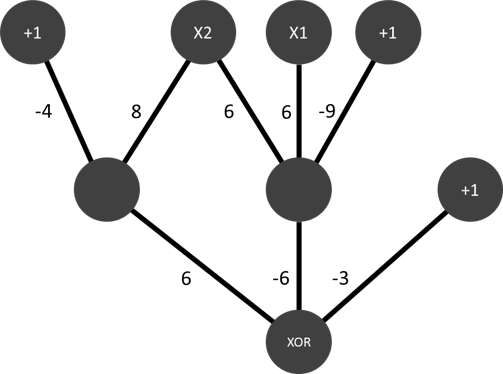$b1 = -9 + 6 * x1 + 6 * x2$

$b2 = -4 + 8 * x1 + 8 * x2$

for x1=0, x2=0

$b1=-9$

$b2=-4$

$b(x)$ is negative, $b(x)$ is 0

### ii) AND Problem¶

We can contintue with the AND problem.

X1 X2 b(x)
0 0 0
0 1 0
1 0 0
1 1 1

We can put everything together into one network graph

### iii) (X1 XOR X2) AND X3¶

The network including the AND would look like the following:

In :
fig = Image(filename=('img/p2.png'))
fig

Out: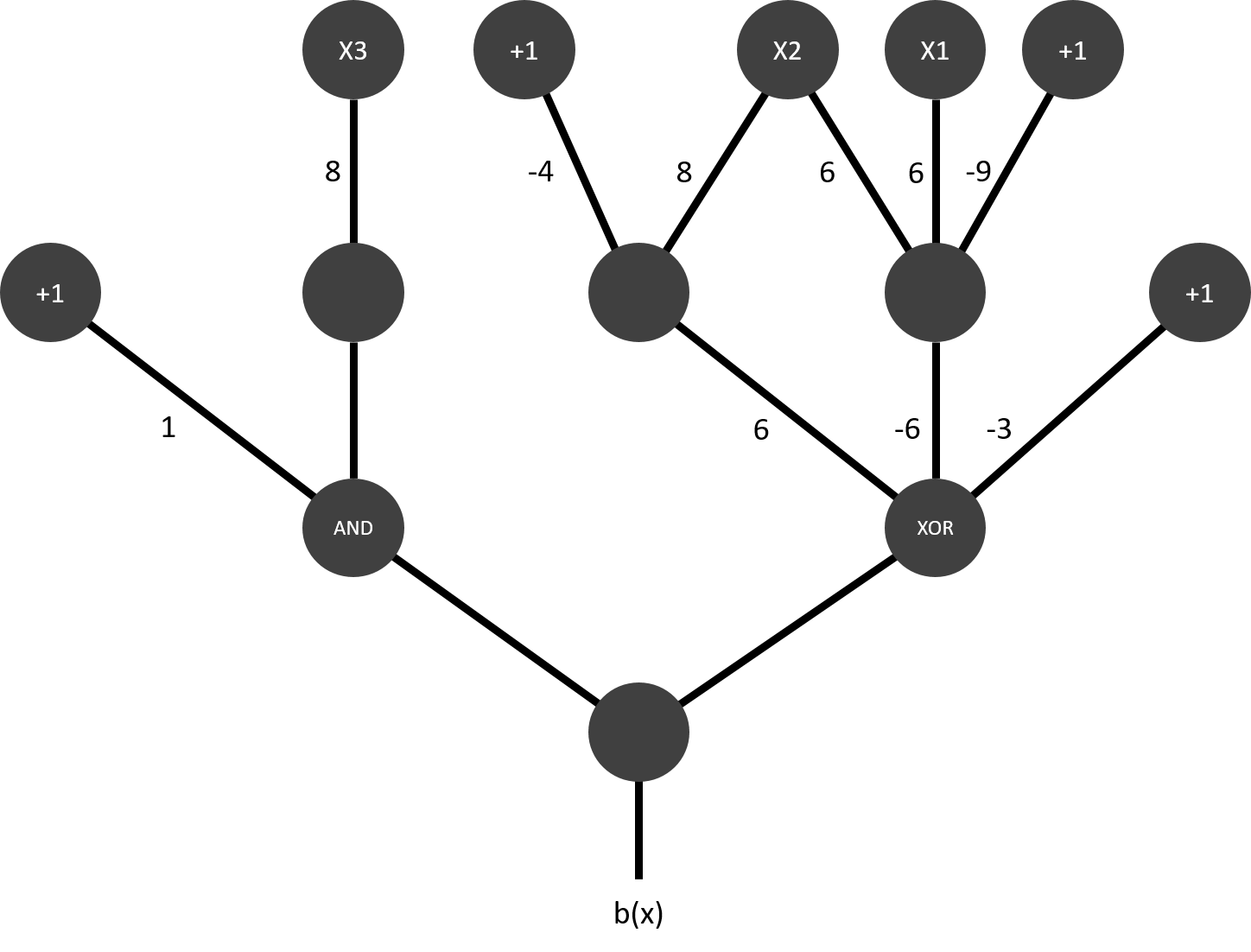The overall truth table looks like this:

X1 X2 X3 b(x)
0 0 0 0
0 0 1 0
0 1 0 0
0 1 1 1
1 0 0 0
1 0 1 1
1 1 0 0
1 1 1 0

# Problem 3 (25%)¶

Determine the value of number e = 2.7183… to 6 decimal places using Taylor expansion. Export the TensorBoard graph of your process. Perform similar calculation using expression for e as lim┬(n → ∞)⁡〖〖(1+ 1/n)〗^n 〗. Again export the TensorBoard graph of you process. Provide working code for both approaches.

Note: Alas, I was first working on the problemset uploaded to canvas (the one with the Fibonacci sequence). I did realize too late, that the problem send by mail was different. Please send us a short info mail if such a thing happens in the future? I lost quite some time on the wrong document.

### i) Taylor expansion (Python)¶

We're starting with the first approach. As stoping parameter we're defining the stop value as: 2.718055556. Before we work in Tensorflow we're working in Python.

In :
# Load libraries
import tensorflow as tf
import numpy as np
import matplotlib.pylab as plt

In :
# Parameters
i = 1
tay_sum = 0
break_value = 10

# While loop
while True:

# Stop condition
break

# Print output
i += 1
if i > break_value:
break


2.0
2.0
2.25
2.25
2.37037037037
2.37037037037
2.44140625
2.44140625
2.48832
2.48832
2.52162637174
2.52162637174
2.54649969704
2.54649969704
2.56578451395
2.56578451395
2.58117479171
2.58117479171
2.5937424601
2.5937424601


### ii) Taylor expansion (Tensorflow)¶

Next we're working in Tensorflow on the same problem.

In :
# Parameters
stop_value = 2.718055556
break_value = 10
n = 1.0
i = 1
f = [tf.constant(0), tf.constant(1)]

# Function
def after_point(x):
s = str(x)
if not '.' in s:
return 0
return len(s) - s.index('.') - 1

# Series
while True:
print(("n:{0}, sum of series: {1})").format(i, '%.20f'%(n)))
if n >= stop_value:
break
n = float((1+1/n) ** n)
print(after_point(n))
i += 1
if i > break_value:
break

n:1, sum of series: 1.00000000000000000000)
1
n:2, sum of series: 2.00000000000000000000)
2
n:3, sum of series: 2.25000000000000000000)
11
n:4, sum of series: 2.28731983699441876468)
11
n:5, sum of series: 2.29238379904733502457)
11
n:6, sum of series: 2.29306172686631937196)
11
n:7, sum of series: 2.29315231843019340374)
11
n:8, sum of series: 2.29316442125037456279)
11
n:9, sum of series: 2.29316603810625929682)
11
n:10, sum of series: 2.29316625410646279803)
11

In :
# Output to tensorflow
with tf.Session() as sess:
output = sess.run(f)
print(output)

tf.summary.FileWriter ("p3", sess.graph)

[0, 1]

Out:
<tensorflow.python.summary.writer.writer.FileWriter at 0x7effa1327e10>

### Get graph¶

With the following command we can get the graph:

tensorboard --logdir="p3"

In :
fig = Image(filename=('/home/tim/img/p3.jpg'))
fig

Out: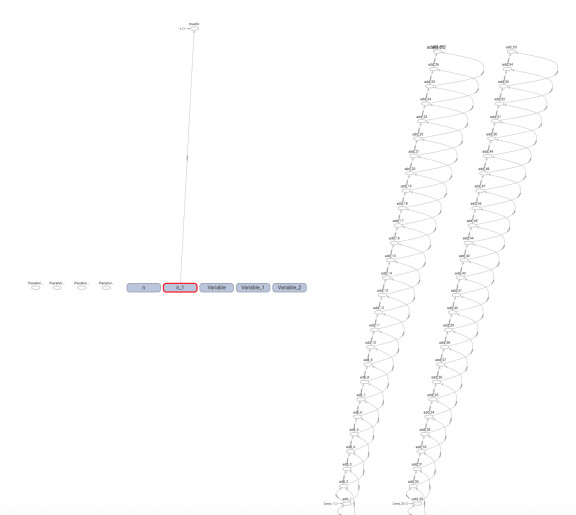# Problem 4 (15%)¶

When I tried running code on page 63 om my notes for lecture 10, the resulting TensorBoard graph was not entirely identical with the graph on page 64. Please fix the code on page 63 in order to produce the graph identical to the graph on page 64.

### Fix code¶

Below you can find the fixed code:

In :
import tensorflow as tf

with tf.name_scope("Scope_A"):
b = tf.multiply(a, 3, name="A_mul")

with tf.name_scope("Scope_B"):
d = tf.multiply(c, 6, name="B_mul")

with tf.name_scope("Output"):

writer = tf.summary.FileWriter('p4', graph=tf.get_default_graph())
writer.close()


### Get graph¶

With the following command we can get the graph:

tensorboard --logdir="p4"

In :
fig = Image(filename=('/home/tim/img/p4.png'))
fig

Out: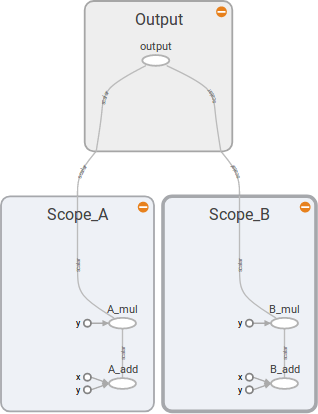# Problem 5 (20%)¶

Please examine attached Jupyter notebook 2_linear_regression.ipynb. As you are running its cells, the notebook will complain about non-existent API calls. This notebook was written in an earlier version of TensorFlow API and some calls changed their names. Fix all code by replacing older calls with calls in TF 1.4 Uncomment all optional (print) lines. Provide a copy of this notebook with all intermediate results and the image of TensorFlow graph as captured by the TensorBoard.

### Fix code¶

Below is the fixed code:

In :
# Import tensorflow and other libraries.
from __future__ import absolute_import
from __future__ import division
from __future__ import print_function

import tensorflow as tf

import numpy as np
import math

get_ipython().magic(u'matplotlib inline')
import pylab

In :
sess = None
def resetSession():
tf.reset_default_graph()
global sess
if sess is not None: sess.close()
sess = tf.InteractiveSession()

In :
resetSession()

print(tf.__version__)

# Create input data using NumPy. y = x * 0.1 + 0.3 + noise
x_train = np.random.rand(100).astype(np.float32)
noise = np.random.normal(scale=0.01, size=len(x_train))
y_train = x_train * 0.1 + 0.3 + noise

# Uncomment the following line to plot our input data.
pylab.plot(x_train, y_train, '.')

1.4.0

Out:
[<matplotlib.lines.Line2D at 0x7f1230171390>]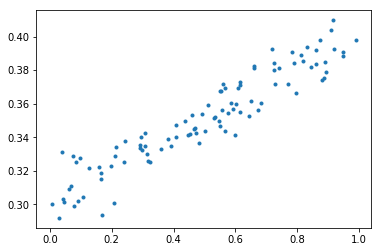In :
# Create some fake evaluation data
x_eval = np.random.rand(len(x_train)).astype(np.float32)
noise = np.random.normal(scale=0.01, size=len(x_train))
y_eval = x_eval * 0.1 + 0.3 + noise

In :
# Build inference graph.
# Create Variables W and b that compute y_data = W * x_data + b
W = tf.Variable(tf.random_normal(), name='weights')
b = tf.Variable(tf.random_normal(), name='bias')

# Uncomment the following lines to see what W and b are.
print(W)
print(b)

# Create a placeholder we'll use later to feed x's into the graph for training and eval.
# shape=[None] means we can put in any number of examples.
# This is used for minibatch training, and to evaluate a lot of examples at once.
x = tf.placeholder(shape=[None], dtype=tf.float32, name='x')

# Uncomment this line to see what x is
print(x)

# This is the same as tf.add(tf.mul(W, x), b), but looks nicer
y = W * x + b

<tf.Variable 'weights_2:0' shape=(1,) dtype=float32_ref>
<tf.Variable 'bias_2:0' shape=(1,) dtype=float32_ref>
Tensor("x_2:0", shape=(?,), dtype=float32)


At this point, we have:

• x_train: x input features
• y_train: observed y for each x that we will train on
• x_eval, y_eval: Same as above, but a smaller set that we will not train on, and instead evaluate our effectiveness.
In :
# Write the graph so we can look at it in TensorBoard
# https://www.tensorflow.org/versions/r0.12/how_tos/summaries_and_tensorboard/index.html
#sw = tf.train.SummaryWriter('summaries/', graph=tf.get_default_graph())
sw = tf.summary.FileWriter('summaries/', graph=tf.get_default_graph())


### Get graph¶

With the following command we can get the graph:

tensorboard --logdir="summaries"

In :
fig = Image(filename=('/home/tim/img/p5.png'))
fig

Out: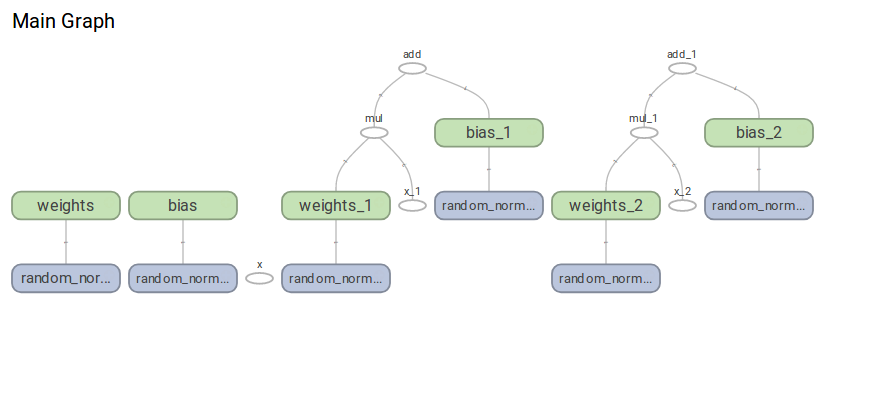In :
# Create a placeholder we'll use later to feed the correct y value into the graph
y_label = tf.placeholder(shape=[None], dtype=tf.float32, name='y_label')
print (y_label)

Tensor("y_label:0", shape=(?,), dtype=float32)

In :
# Build training graph.
loss = tf.reduce_mean(tf.square(y - y_label))  # Create an operation that calculates loss.
optimizer = tf.train.GradientDescentOptimizer(0.5)  # Create an optimizer.
train = optimizer.minimize(loss)  # Create an operation that minimizes loss.

# Uncomment the following 3 lines to see what 'loss', 'optimizer' and 'train' are.
print("loss:", loss)
print("optimizer:", optimizer)
print("train:", train)

loss: Tensor("Mean:0", shape=(), dtype=float32)
op: "NoOp"


In :
# Create an operation to initialize all the variables.
#init = tf.initialize_all_variables()
init = tf.global_variables_initializer()
print(init)
sess.run(init)

name: "init"
op: "NoOp"
input: "^weights/Assign"
input: "^bias/Assign"
input: "^weights_1/Assign"
input: "^bias_1/Assign"
input: "^weights_2/Assign"
input: "^bias_2/Assign"


In :
# Uncomment the following line to see the initial W and b values.
print(sess.run([W, b]))

[array([-1.59608626], dtype=float32), array([-0.61943322], dtype=float32)]

In :
# Uncomment these lines to test that we can compute a y from an x (without having trained anything).
# x must be a vector, hence  not just 3.
x_in = 
sess.run(y, feed_dict={x: x_in})

Out:
array([-5.40769196], dtype=float32)
In :
# Calculate loss on the evaluation data before training
def eval_loss():
return sess.run(loss, feed_dict={x: x_eval, y_label: y_eval})
eval_loss()

Out:
3.3503497
In :
# Track of how loss changes, so we can visualize it in TensorBoard
#tf.scalar_summary('loss', loss)
tf.summary.scalar('loss', loss)
#summary_op = tf.merge_all_summaries()
summary_op = tf.summary.merge_all()

In :
# Perform training.
for step in range(201):
# Run the training op; feed the training data into the graph
summary_str, _ = sess.run([summary_op, train], feed_dict={x: x_train, y_label: y_train})
# Uncomment the following two lines to watch training happen real time.
if step % 20 == 0:
print(step, sess.run([W, b]))

0 [array([-0.5965234], dtype=float32), array([ 1.14253831], dtype=float32)]
20 [array([-0.16607681], dtype=float32), array([ 0.44172648], dtype=float32)]
40 [array([ 0.01957429], dtype=float32), array([ 0.34391209], dtype=float32)]
60 [array([ 0.0746385], dtype=float32), array([ 0.31490028], dtype=float32)]
80 [array([ 0.09097057], dtype=float32), array([ 0.30629537], dtype=float32)]
100 [array([ 0.09581465], dtype=float32), array([ 0.30374315], dtype=float32)]
120 [array([ 0.09725142], dtype=float32), array([ 0.30298617], dtype=float32)]
140 [array([ 0.09767753], dtype=float32), array([ 0.30276167], dtype=float32)]
160 [array([ 0.09780393], dtype=float32), array([ 0.30269507], dtype=float32)]
180 [array([ 0.09784143], dtype=float32), array([ 0.30267531], dtype=float32)]
200 [array([ 0.09785255], dtype=float32), array([ 0.30266944], dtype=float32)]

In :
# Uncomment the following lines to plot the predicted values
pylab.plot(x_train, y_train, '.', label="target")
pylab.plot(x_train, sess.run(y, feed_dict={x: x_train, y_label: y_train}), label="predicted")
pylab.legend()

Out:
<matplotlib.legend.Legend at 0x7f120ff6e690>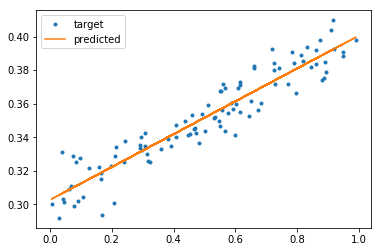In :
# Check accuracy on eval data after training
eval_loss()

Out:
0.00011943727

### Get graph¶

With the following command we can get the loess graph:

tensorboard --logdir="summaries"

In :
fig = Image(filename=('/home/tim/img/p5_2.png'))
fig

Out: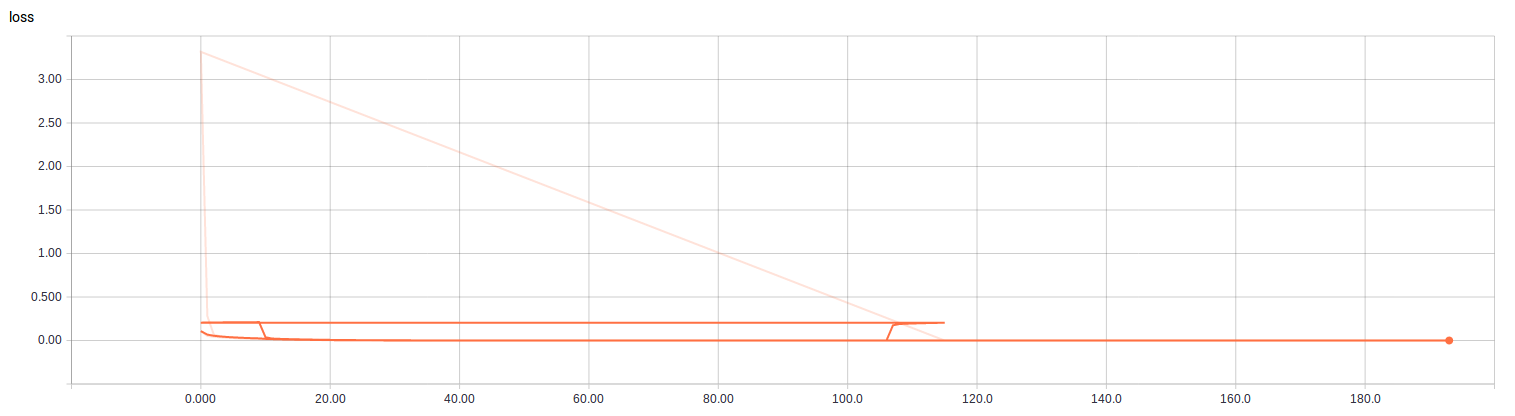In :
def predict(x_in): return sess.run(y, feed_dict={x: [x_in]})

In :
# Save the model
saver = tf.train.Saver()

saver.save(sess, '/home/tim/my_checkpoint.ckpt')

Out:
'/home/tim/my_checkpoint.ckpt'
In :
# Current prediction
predict(3)

Out:
array([ 0.59622705], dtype=float32)
In :
# Reset the model by running the init op again
sess.run(init)

In :
# Prediction after variables reinitialized
predict(3)

Out:
array([ 6.26759624], dtype=float32)
In :
saver.restore(sess, '/home/tim/my_checkpoint.ckpt')

INFO:tensorflow:Restoring parameters from /home/tim/my_checkpoint.ckpt

In :
# Predictions after variables restored
predict(3)

Out:
array([ 0.59622705], dtype=float32)